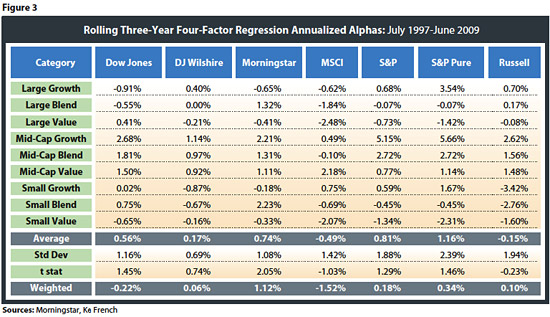# Can Indexes Generate Alpha?

February 17, 2010

The excess return of the index (which is defined as the return of the index for the month minus the risk-free rate for the month) is regressed against a market beta factor (defined as the return on the market minus the risk-free rate), a value factor (or HML, defined as the return on value stocks minus the return on growth stocks), a size factor (or SMB, defined as the return on small stocks minus the return on big stocks), and a momentum factor (based on the six value-weight portfolios formed on size and prior return, the average return on the two high prior-return portfolios minus the average return on the two low prior-return portfolios). The four-factor regression equation is:

Rindex – Rf = ?index + ?index (Rmarket – Rf) + ?SMB(SMB) + ?HML(HML) + ?MOM(Momentum) + ?asset

where Rindex is the return on the index, Rf is the risk-free rate, ?index is the alpha of the index, ?index is the index’s beta with respect to the market, Rmarket is the return of the market, ?SMB is the index’s beta with respect to the “large” factor (SMB), ?HML is the index’s beta with respect to the “value” factor (HML), ?MOM is the index’s beta with respect to the “momentum” factor (MOM), and ?asset is the error term. All monthly alpha estimates are annualized for comparative purposes. For those readers not familiar with four-factor regression approach, see Fama and French  and Carhart .

Cremers, Petajisto and Zitzewitz  have noted that the standard Fama-French (three-factor) and Carhart (four-factor) regression models can produce statistically significant nonzero alphas for passive indexes primarily from the disproportionate weight the Fama-French factors place on small value stocks (which have performed well). While Cremers et al. introduce regression factors that outperform standard models in their paper, the traditional four-factor estimates are used for this research, due to their widespread use and acceptance. While the reader may contend that an index (i.e., a broad, well-diversified and passive portfolio) should not have an alpha component by definition, using a method that is widely employed to determine alpha for active managers (the four-factor Carhart approach with the traditional Fama-French factors) can in fact generate one.

For the analysis, regressions are based on rolling three-year periods, which consist of 36 months of historical data. Rolling periods are used versus a single period to account for potential changing market exposures of the indexes over time, as well as to make the analysis less dependent on the period studied. For example, if an index methodology did very well the first and last months of the test look-back period, it may appear that it generated alpha during the entire study, despite the fact it dramatically under-performed the months in between. Also, the average implied holding period for equity mutual funds is approximately three years based on a current redemption rate of 30 percent per year [ICI 2009], which makes the rolling three-year regression method more relevant to how investors actually invest in equity mutual funds.

Seven different index methodologies are considered for the analysis: Dow Jones, Dow Jones Wilshire, Morningstar, MSCI, Standard & Poor’s, Standard & Poor’s Pure and Russell, with the actual underlying tested indexes listed in Appendix II. The time period for the analysis is from July 1997 until June 2009, which is the longest period for which data was available for the different indexes (all nine domestic styles for each of the seven different providers). Using the same period for all methodologies allows for a more relative comparison than using the entire period of data available for each index. The total number of three-year test periods is 109.

Results

The average four-factor regression alphas for each of the indexes for each style are included in Figure 3, as well as the average alpha across styles, standard deviation of alphas across styles and the average alpha across the styles’ t statistics for each methodology. Information on the weighted outperformance is also included, where the respective alphas are weighted by the total net assets invested in all passive index funds and ETFs as of June 30, 2009. This number reflects how investors actually invest in index funds at the total asset level, versus the simple average that is used for statistical significance purposes for each methodology.